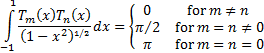/

### Chebyshev Polynomials

The Chebyshev polynomial of degreeis denoted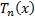. It is defined as: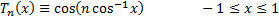Chebyshev polynomials satisfy the following relationships and recursion formulae:

Recursion formulae: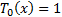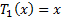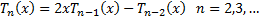Zeros, exactlydistinct zeros in the interval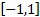, as follows: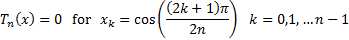Extrema, exactly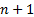real extrema in the interval, as follows: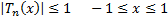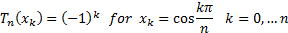Orthogonality: